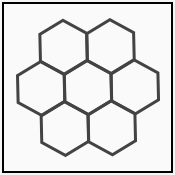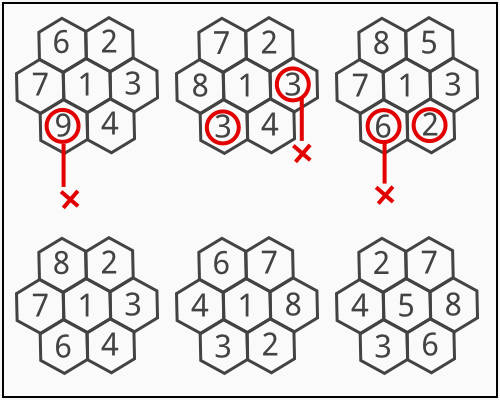### Problem Statement

John and Brus are interested in a new game called "Hexagon Flower". The rules are simple. You are given a flower formed by seven hexagons arranged as follows:The objective of the game is to place a number in each hexagon of the flower such that all of the following conditions are satisfied:

• Each number is an integer between 1 and n*2, inclusive.
• Each number is distinct.
• For every pair of adjacent hexagons, if the numbers placed in them are a and b, then a%n != b%n.

Given n, return the total number of distinct solutions. Two solutions are considered the same if you can rotate one to form the other.

For example, if n = 4 then:The top three placements are not valid. The other three placements are valid, but the first two among them are considered equal since one can be rotated to become the other.

### Definition

 Class: TheHexagonsDivOne Method: count Parameters: int Returns: long Method signature: long count(int n) (be sure your method is public)

### Constraints

-n will be between 1 and 150, inclusive.

### Examples

0)

 `3`
`Returns: 0`
 There are not enough numbers to fill the flower with.
1)

 `4`
`Returns: 256`
2)

 `8`
`Returns: 3458560`
3)

 `20`
`Returns: 11193888000`

#### Problem url:

http://www.topcoder.com/stat?c=problem_statement&pm=10702

#### Problem stats url:

http://www.topcoder.com/tc?module=ProblemDetail&rd=14144&pm=10702

vexorian

#### Testers:

PabloGilberto , ivan_metelsky , Chmel_Tolstiy

#### Problem categories:

Graph Theory, Simulation